Number Sequence Involving Fraction  by  Alice Akinyi

1

DIVISION OF A DECIMAL  NUMBER   BY A WHOLE NUMBER

What is Division?

[resource: 6156, align: left]

•Division is one of the four basic operations in arithmetic (mathematics) apart from addition(+), subtraction (-), and multiplication (×).
•Division means splitting into equal parts or groups. It's the result of fair sharing. For example;
•Kamau has 7.5 kg of sugar to share among his 5 siblings.How many kilograms of sugar will each sibling get?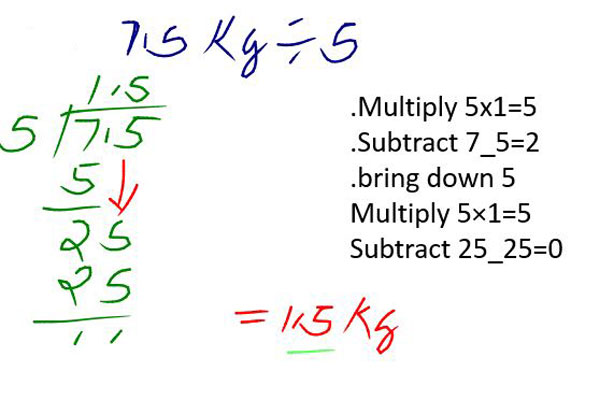NOTE THE FOLLOWING

•In division we have;
•Dividend which is the number to be divided by the divisor.
•Divisor is the number we use  to divide another  Number which we call dividend.
•The answer we get after dividing dividend by the divisor is called quotient
•In division the rule is ;
•Divide into
•Multiply
•Subtract
•Bring down
•Place the decimal point directly above the decimal point in the dividend, so it lines up with the answer.

Therefore;if Kamau has 7.5kg of sugar to share among his 5 siblings,each sibling will get?
Multiply the quotient by the divisor to get the dividend and to confirm if your answer is correct.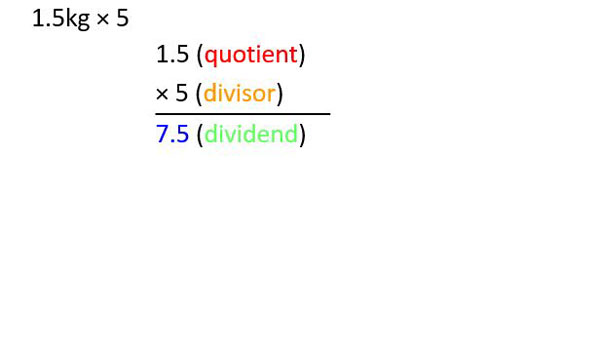Work out the following examples

Example 1

•Ruth has Shs. 24.50 to share between her two sons. How much will each son get?
•Solution;
•Shs.24.50 is the dividend while 2 is the divisor.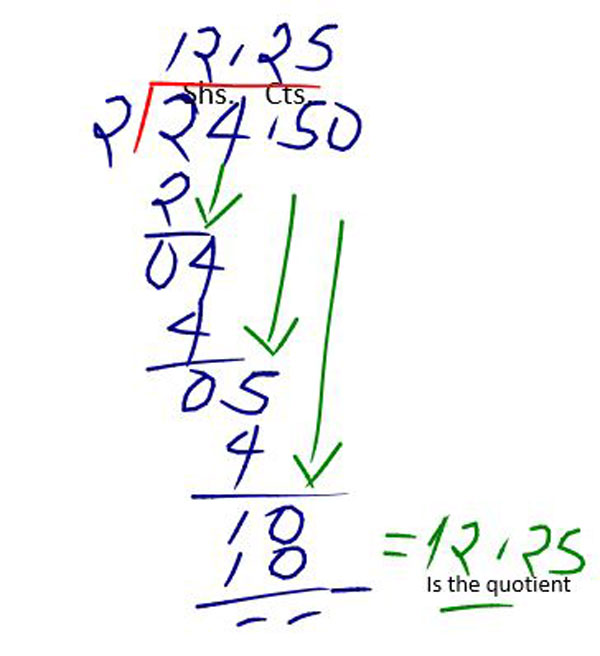Example 2

Divide 9.396 by 9
•9.396 is the dividend while 9 is the divisor.
•Use long division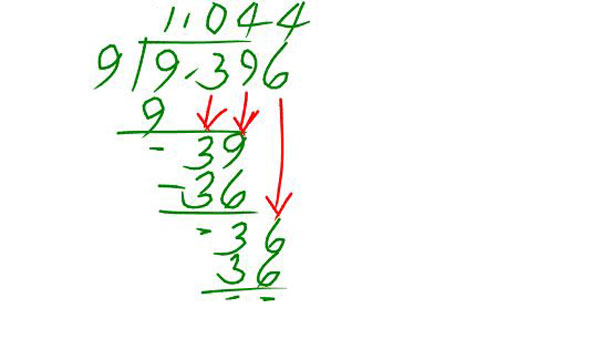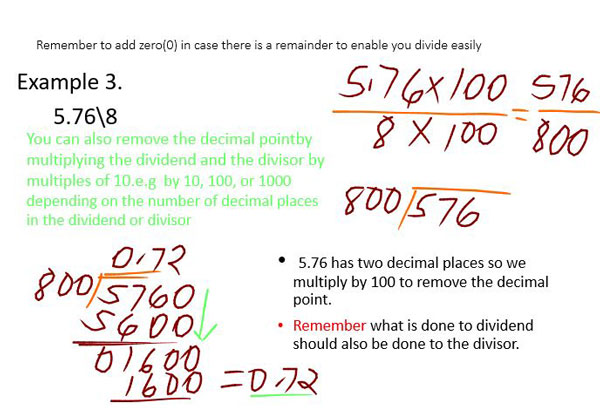Exercise

•Work out the following
1.2.495 divide by 5
2.23.4 divide by 8
3.12.72 divide by 6
4.Rose had a string of length 16.1meters long.she decided to cut it into 7 equal pieces.How long was each piece?
5.A cook had a bag of rice weighing 17.75kg. He shared the rice equally between 50 students, how much did each student get?